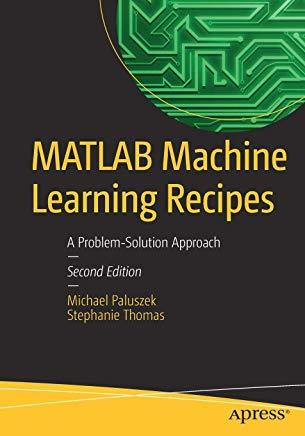### MATLAB Machine Learning Recipes: A Problem-Solution Approach### Huge savings for students

Each student receives a 50% discount off of most books in the HSG Book Store. During class, please ask the instructor about purchase details.
 List Price: \$29.99 Price: \$15.00 You Save: \$15.00
4Harness the power of MATLAB to resolve a wide range of machine learning challenges. This book provides a series of examples of technologies critical to machine learning. Each example solves a real-world problem. All code in MATLAB Machine Learning Recipes: A Problem-Solution Approach is executable. The toolbox that the code uses provides a complete set of functions needed to implement all aspects of machine learning. Authors Michael Paluszek and Stephanie Thomas show how all of these technologies allow the reader to build sophisticated applications to solve problems with pattern recognition, autonomous driving, expert systems, and much more.
What you'll learn:
• How to write code for machine learning, adaptive control and estimation using MATLAB
• How these three areas complement each other
• How these three areas are needed for robust machine learning applications
• How to use MATLAB graphics and visualization tools for machine learning
• How to code real world examples in MATLAB for major applications of machine learning in big data
Who is this book for: The primary audiences are engineers, data scientists and students wanting a comprehensive and code cookbook rich in examples on machine learning using MATLAB.
Apress